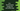# Find the factorial in C++ using a for and while loop## Introduction :

We can find the factorial of a number using a loop. The factorial of a number is the product of all numbers from 1 to that number. Finding out the factorial using a loop like for or while loop is easy. In this post, I will show you how to find the factorial of a user given number in C++ using a loop.

### Example 1 : C++ program to find factorial using a for loop :

To find the factorial, we will run one for loop from 1 to that number. On each iteration, we will multiply the numbers to get the final factorial. Below is the complete program :

``````#include <iostream>
using namespace std;

int main()
{
int number, factorial = 1;

cout << "Enter a number :" << endl;
cin >> number;

for (int i = 1; i <= number; i++)
{
factorial *= i;
}

cout << "Factorial : " << factorial << endl;

return 0;
}``````

Here, the program will ask the user to enter a number. It reads the number and stores it in variable number. We are running one for loop from i = 1 to i = number. We also have initialized another variable factorial as 1. This variable is to hold the final factorial. Inside the loop, on each iteration, we are multiplying the current value of i with factorial. Once the loop will end, factorial will hold the factorial of the given number. We can also run this loop from i = 2 i.e. we can multiply all numbers from i = 2 to i = number. Multiplying a number by 1 willl result the same.

Sample outputs of the above program :

``````Enter a number :
4
Factorial : 24

Enter a number :
5
Factorial : 120``````

### Example 2 : C++ program to find factorial using a while loop :

Using a while loop is similar to for loop. The only difference is that, while loop checks one condition and execute its body. It will keep executing until the condition is true. In our case, we will keep decrementing the user given number by 1 and on each step, we will keep the current value multiplying to the final factorial. Below is the complete program :

``````#include <iostream>
using namespace std;

int main()
{
int number, factorial = 1;

cout << "Enter a number :" << endl;
cin >> number;

while(number > 1)
{
factorial *= number;
number --;
}

cout << "Factorial : " << factorial << endl;

return 0;
}``````

The while loop runs if the value of number is more than 1. On each step, we are multiplying its value to the variable factorial and decrementing it by 1. For example, if the value of number is 5, it will run for 5, 4, 3, 2 and the value of factorial will be 5 * 4 * 3 * 2 i.e. the factorial of 5. We don’t have to multiply it with 1 as that will be same. The benifit of this approach is that we don’t have to initialize one extra variable i like we did with the for loop. It is more efficient than the for loop approach in terms of space complexity. It will produce the same output. You can also use any other loop like do while to find the factorial of a number in C++. It will be a similar way to do that. You can try that and drop one comment below :).

### Conclusion :

I hope that you have learned how to find factorial in C++. Both ways are equally useful. You can use any of these methods. If you have any queries, don’t hesitate to drop one comment below.